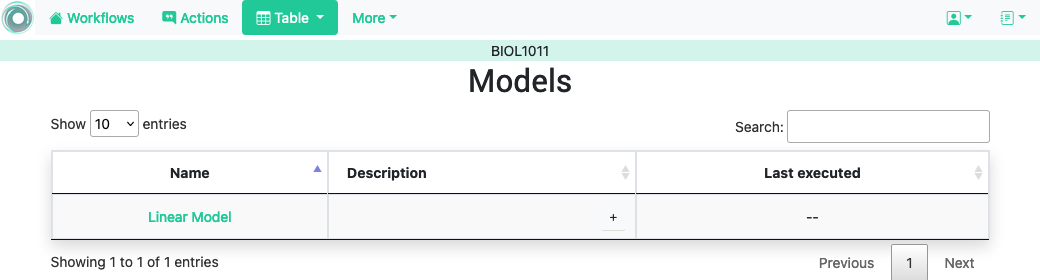# 5.26. Write your own data processing code¶

## 5.26.1. Example: A Predictive Model¶

Suppose that your favorite data analyst has processed the data set and created a predictive model that estimates the score of the final exam based on the value of the column Contributions applying the following linear equation:

```final exam score = 3.73 * Contributions + 25.4
```

You would like to incorporate this model to the workflow and use the predicted final exam score as another column to create conditions and personalize content. One way to achieve this is by creating a plugin that given the two coefficients of a linear model (in the example 3.73 and 25.4) returns a new data set with a column with the values obtained using the corresponding equation. In order for the plugin to comply with the requirements, one possible definition would be:

```# -*- coding: utf-8 -*-

from typing import Dict, Optional

import pandas as pd

class_name = 'LinearModel'

"""Plugin to execute the linear model y = 3.73 * Contribution + 25.4

The result is stored in column 'Final Exam Predict'
"""

def __init__(self):
"""Initialize all the fields."""
super().__init__()
self.name = 'Linear Model'
self.description_text = "Obtain a prediction of the final exam score."
self.input_column_names = ['Contribution']
self.output_column_names = ['Final Exam Predict']

def run(self, data_frame: pd.DataFrame, parameters: Optional[Dict] = dict):
"""
Parse the parameters to guarantee that they were correct, and if so,
returns the dataframe with the resulting linear model.

:param data_frame: Input data for the plugin
:param parameters: Dictionary with (name, value) pairs.
:return: a Pandas data_frame to merge with the existing one
"""

new_dataframe = pd.DataFrame(3.73 * data_frame['Contribution'] + 25.4)
new_dataframe.columns = self.output_column_names

return new_dataframe
```

The steps to run the model are:

• Click in the Table icon in the top menu and select the option Run model. The table will include those models ready for execution.• Click in the name of the model. The next screen contains four tabs:

Input columns to transformation

Select the columns to use as input data for the model.

Columns to store the result

Provide a set of columns to store the result of running the model and one key column to merge the results with the existing table (mandatory).

Parameters

A set of parameters to execute the model (could be empty).

Description

A more detailed description of what the model does.

• Select the appropriate elements and click on the Run button above the form.

• The model is executed in the background (it may take some time to execute) and the result is merged into the workflow table.